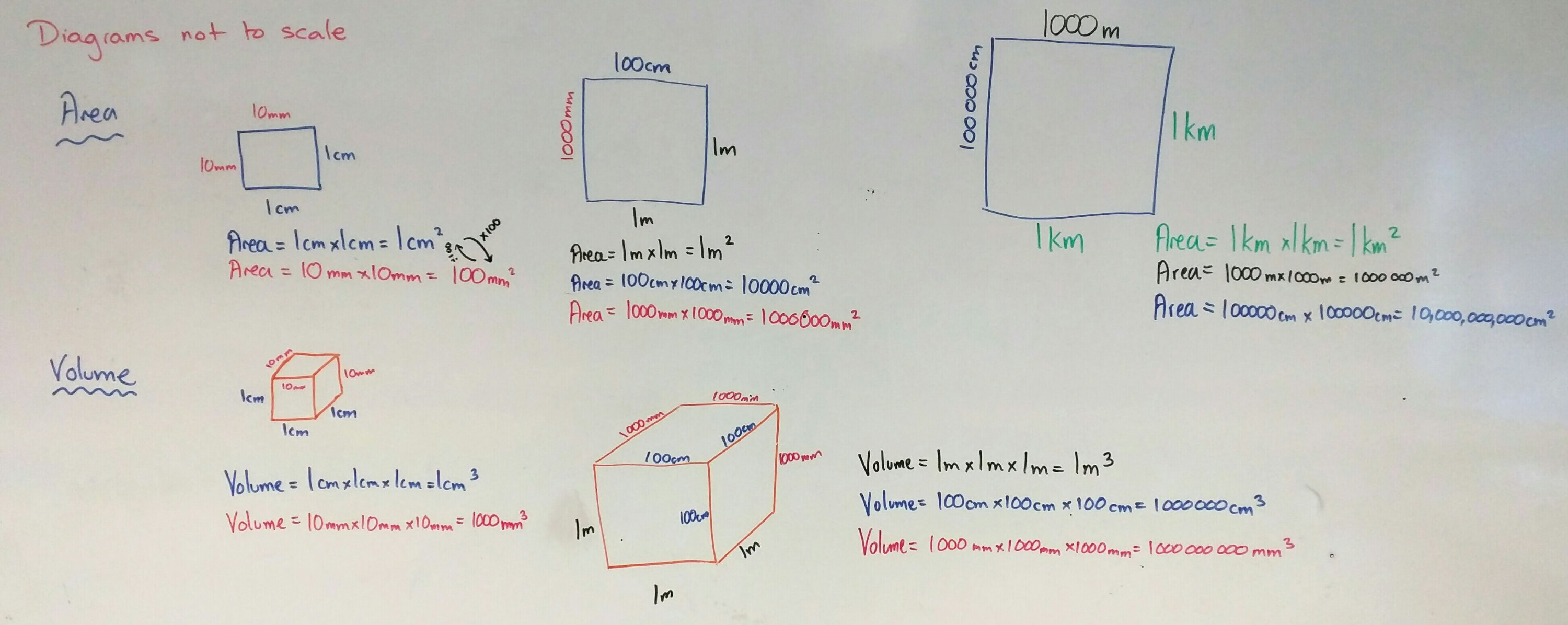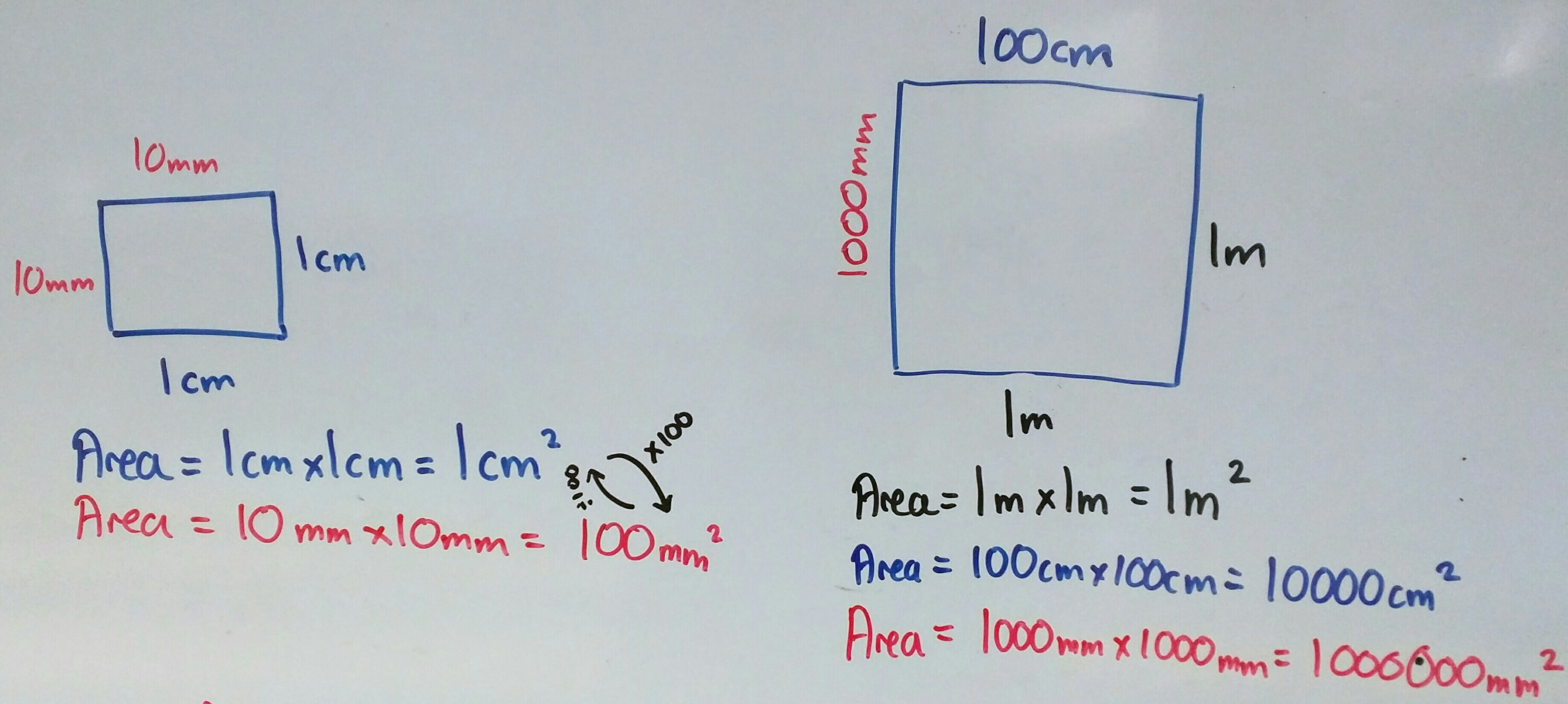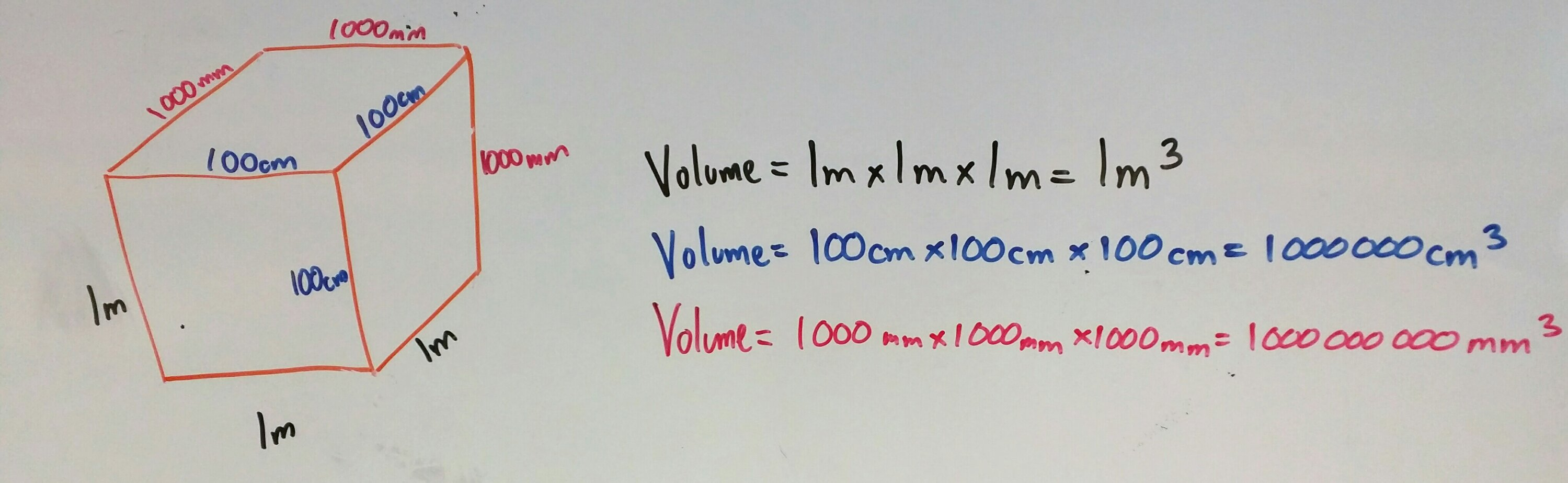346. Area & Volume conversion

This is a quick post on how I teach metric unit conversion for area and volume. All you need is a big whiteboard and coloured board pens.Start by stressing that all diagrams are not to scale/accurate.

Two colours

1. Draw a square on the board
2. Pen colour 1: Label it as 1cm
3. What is the area? Show the calculation
4. What is 1cm in mm?
5. Pen colour 2: Label it as 10mm
6. What is the area in mm? Show the calculation
7. What is the scale factor between the sides? the area? why?Three colours

1. Draw a square on the board
2. Pen colour 3: Label it as 1m
3. What is the area? Show the calculation
4. What is 1m in cm?
5. Pen colour 2: Label it as 100cm
6. What is the area in cm? Show the calculation
7. What is the scale factor between the sides? the area? why?
8. Repeat in pen colour 1 for mm

Four colours

Well not actually four colours – pens 2,3 & 4 only. Repeat the process for kilometres to metres and centimetres.

Volume – same process, just three dimensionsWhy all the colours?

By coding each unit of measurement with a colour students can see the progression of the calculations and the links between area/volume and scale factor. After all, an okay mathematician can reproduce memorised facts, but a great mathematician doesn’t need to memorise – they understand where the calculations came from.

345. Practical percentage skills

It’s perfectly obvious that fluency in the use of multiplication tables directly impacts students ability to divide. This grows into confidence with algebra and reverse operations. Students are able to see the links between the concepts. Our understanding of the importance of such skills is part of the success of programmes such as TTRockstars and Numeracy Ninjas.

Why is it then that so many textbooks, websites and resource banks keep the manipulation of percentages as separate skills sets? Percentage increase / Percentage change / Reverse percentages. We know that when concepts overlap, fluency increases when these links are pursued. So that’s what I set out to do.

I have a bright Year 8 class and started working on percentages with them. It didn’t take much to have them confident using equivalent decimal multipliers to find percentages of amounts. Using a multiplier for increase/decrease was a walk in the park. Then finding percentage change came up. Over the years I’ve seen a lot of students get very confused with half remembered methods:

“Which do I take away?”

“What number do I divide by?”

“Is this calculation the right way around?”

I tend to teach new value divided by old value and interpret the answer. It got me thinking – why am I teaching them this? They can increase by a percentage using a multiplier, why can’t they rearrange their working to find the actual percentage? Same goes for reversing a percentage.

After a good discussion, I used this worksheet to recap and develop their skills: